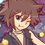# Inequalities & Functional Equations (Thailand Math POSN 3rd round)

Write a full solution.

Inequalities

Theorems allowed to use: basic inequalities, AM-GM-HM, Cauchy Schwarz, Holder, Weighted AM-GM, Jensen, Power Mean, Chebyshev, Rearrangement, Weirstrass, Bernoulli

Comments by professor: These 2 problems can be solved using AM-GM and Cauchy Schwarz only. Maybe you can try it!

1.) Let $a,b,c$ be sides of triangle, $\displaystyle s = \frac{a+b+c}{2}$, prove that for all positive integers $n$

$\displaystyle \frac{a^{n}}{b+c}+\frac{b^{n}}{c+a}+\frac{c^{n}}{a+b} \geq \left(\frac{2}{3}\right)^{n-2}s^{n-1}$

2.) Let $0 < x_{1} \leq x_{2} \leq \dots \leq x_{2558}$ such that $\displaystyle \sum\limits_{k=1}^{2558} \frac{1}{1+x_{k}} = 1$, prove that

$\displaystyle \sum\limits_{k=1}^{2558}\sqrt{x_{k}} \geq 2557\left(\sum\limits_{k=1}^{2558}\frac{1}{\sqrt{x_{k}}}\right)$

Functional Equations

1.) Find all functions $f: \mathbb{R} \rightarrow \mathbb{R}$ that satisfy for all $x,y,z \in \mathbb{R}$.

$\displaystyle \frac{f(xy)}{2}+\frac{f(xz)}{2}-f(x)f(yz) \geq \frac{1}{4}$

2.) Find all strictly monotonic functions $f: \mathbb{R} \rightarrow \mathbb{R}$ that satisfy for all $x \in \mathbb{R}$.

$f(x)+f^{-1}(x) = 2x$

This note is part of Thailand Math POSN 3rd round 2015Note by Samuraiwarm Tsunayoshi
6 years, 3 months ago

This discussion board is a place to discuss our Daily Challenges and the math and science related to those challenges. Explanations are more than just a solution — they should explain the steps and thinking strategies that you used to obtain the solution. Comments should further the discussion of math and science.

When posting on Brilliant:

• Use the emojis to react to an explanation, whether you're congratulating a job well done , or just really confused .
• Ask specific questions about the challenge or the steps in somebody's explanation. Well-posed questions can add a lot to the discussion, but posting "I don't understand!" doesn't help anyone.
• Try to contribute something new to the discussion, whether it is an extension, generalization or other idea related to the challenge.

MarkdownAppears as
*italics* or _italics_ italics
**bold** or __bold__ bold
- bulleted- list
• bulleted
• list
1. numbered2. list
1. numbered
2. list
Note: you must add a full line of space before and after lists for them to show up correctly
paragraph 1paragraph 2

paragraph 1

paragraph 2

[example link](https://brilliant.org)example link
> This is a quote
This is a quote
    # I indented these lines
# 4 spaces, and now they show
# up as a code block.

print "hello world"
# I indented these lines
# 4 spaces, and now they show
# up as a code block.

print "hello world"
MathAppears as
Remember to wrap math in $$ ... $$ or $ ... $ to ensure proper formatting.
2 \times 3 $2 \times 3$
2^{34} $2^{34}$
a_{i-1} $a_{i-1}$
\frac{2}{3} $\frac{2}{3}$
\sqrt{2} $\sqrt{2}$
\sum_{i=1}^3 $\sum_{i=1}^3$
\sin \theta $\sin \theta$
\boxed{123} $\boxed{123}$

Sort by:

Functional Equations 1) Hints

Step 1: Find $f(0)$.
Step 2: Show that for $f(yz) \geq f(y)$.

Functional Equations 2) Hints
Step 1: Guess what all the possible functions are.
Step 2: Show that if $f(x) = x+c$, then $ff(x) = x + 2c$.

Staff - 6 years, 3 months ago

For 1) you can find that $f(x)$ is bounded above by some value and bounded below by some value. You need to find $f(1)$ though.

- 6 years, 3 months ago

There are likely multiple ways of approaching these functional equations. Here's what i did for 1, without having to find $f(1)$ explicitly.

Let $x = 0, y = 0, z = 0$, we obtain $f(0) ( 1 - f(0 ) \geq \frac{1}{4}$, hence we can conclude that $f(0) = \frac{1}{2}$.

Let $x = x, \neq 0 y = y, z = 0$, we obtain $\frac{ f(xy) } { 2} + \frac{1}{4} - f(x) \times \frac{1}{2} \geq \frac{1}{4}$, or that $f(xy) \geq f(x)$.
But since this is true for all $y$, with $x = x, y = \frac{y}{x}$, we obtain $f( y) \geq f(x)$ for arbitrary $x, y$.. Hence, the only possible function is a constant function, except possibly for $x = 0$.

Now, for any $x \neq 0$, let $x = y = z$, and set $f(x) = a$. We get $a - a^2 \geq \frac {1}{4}$, from which we can conclude that $a = \frac{1}{2}$.

Thus, the only solution is $f(x) = \frac{1}{2} \forall x$.

Note: Yes, it is easy to find $f(1)$ by setting $x = y = z = 1$ to obtain $f(1) = \frac{1}{2}$. There are likely alternative approaches which uses this fact.

Staff - 6 years, 3 months ago

This isn't a full solution.

For first inequality,

Step 1)Use $AM\geq HM$ after writing $b+c=2s-a$

Step 2) The denominator will be in terms of $\frac{1}{a^n}+\frac{1}{b^n}+\frac{1}{c^n}$.Minimize this using Hölder's inequality to the form of $\frac{1}{a}+\frac{1}{b}+\frac{1}{c}$,then again using $AM\geq HM$ write it in terms of $s$ and substitute this minimized value in original denominator,but we should careful while substituting such inequalities.Thus,we need to check equality condition for each and every step.However,it is same for each step$(a=b=c)$. Finally,we arrive at the desired answer.

- 6 years, 3 months ago

Nice set of problems.

For question 2, the initial assumption of "ordered chain" isn't necessary, since the LHS and RHS are both independent of the order of the sequence. I wonder why that put that condition in, rather than simply saying "all positive".

Staff - 6 years, 3 months ago

I think that the condition gives a hint for Chebyshev's Inequality. Not really sure though.

- 6 years, 3 months ago

Can you give solutions especially the functional equations part,

The questions are tricky!

- 6 years, 3 months ago